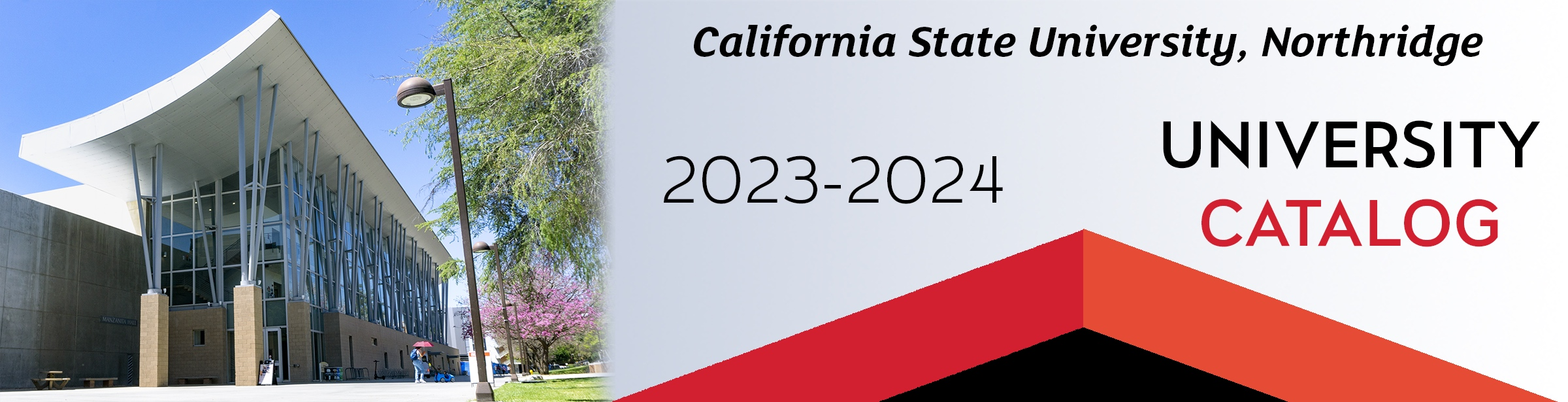All lower division GE completed. Lower division major requirements of Calculus I, Calculus II and Calculus III (MATH 150A, MATH 150B, MATH 250) completed as part of the AS-T in Mathematics.

### Transfer Year 1: First Semester

Course Units
MATH 262 (if not completed prior to transfer) 3
COMP 110/L (if not completed prior to transfer) 4
MATH 320 (satisfies GE B5**) 3
MATH 340 3
MATH 382/L 3
Total 16

### Transfer Year 1: Second Semester

Course Units
MATH 450A 3
MATH 351 3
MATH 360 3
PHYS 220A and PHYS 220AL (if not completed prior to transfer) 4
GE Upper Division** 3
Total 16

### Transfer Year 2: First Semester

Course Units
MATH 462 3
Upper Division MATH Elective* 3
Upper Division MATH Elective* 3
Upper Division MATH Elective* 3
GE Upper Division** 3
Total 15

### Transfer Year 2: Second Semester

Course Units
MATH 493 3
Upper Division MATH Elective* 3
Upper Division MATH Elective* 3
Electives 4
Total 13
 Total units after transfer 60

*Upper division MATH electives – Total of 15 units chosen with an advisor from: MATH 366, MATH 440A, MATH 440B, MATH 450B, MATH 455, MATH 460, MATH 463, MATH 480, MATH 481A, MATH 481B, MATH 481C, MATH 481D, MATH 483, all 500-level MATH except MATH 510-514. MATH 450B or MATH 460 must be taken as one of the elective courses.

* Total of 9 units Upper Division General Education must include 3 units from GE B5 Scientific Inquiry and Quantitative Reasoning (MATH 320 satisfies GE B5), and 6 units from GE Upper Division Section C1 Arts, or GE Upper Division Section C2 Humanities, or GE Upper Division Section D Social Sciences, or GE Upper Division Section F Comparative Cultural Studies. (No more than 3 units from GE Upper Division C and no more than 3 units from GE Upper Division D.) If needed, select UDGE courses that also satisfy: IC: Information Competence, and/or: GE Upper Division Section C3 American History Institutions and Ideals, OR GE Upper Division Section D3 and D4 Constitution of the United States and State and Local Government.

A minimum of 18 units of upper division Mathematics coursework must be completed in residence at CSUN.